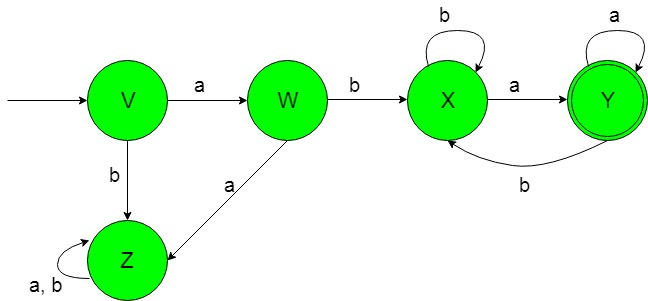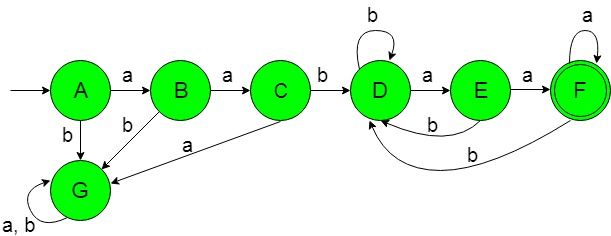# Designing Deterministic Finite Automata (Set 9)

• Difficulty Level : Easy
• Last Updated : 20 Nov, 2019

Prerequisite: Designing finite automata
In this article, we will see some designing of Deterministic Finite Automata (DFA).

Problem-1: Construction of a minimal DFA accepting a set of strings over {a, b} in which {abwa / wε{a, b}*}.
Here ‘w’ is the substring containing alphabets over any number of ‘a’ and ‘b’ ranging from zero to infinity.

Explanation: The desired language will be like:

`L1 = {abεa, abaaaa, ababaaa, abaabbaaa}`

Here as we can see that each string of the above language having constant initial i.e, {ab} and final {a} substring but ‘w’ having a substring over {a, b} whose alphabets ranging from zero to infinity but the below language is not accepted by this DFA because it’s strings are not following the format of string.

`L2 = {baa, aabaa, baabaaa..............}`

The state transition diagram of the desired language will be like below:In the above DFA, the initial state ‘V’ on getting ‘a’ as the input it transits to a state ‘W’ and on getting ‘b’ as the input it transits to a dead state ‘Z’. The state ‘W’ on getting ‘a’ as the input it transits to the dead state ‘Z’ and on getting ‘b’ as the input it transits to a state ‘X’. The state ‘X’ on getting ‘b’ as the input it remains in the state of itself and on getting ‘a’ as the input it transits to a final state ‘Y’.

The final state ‘Y’ on getting ‘a’ as the input it remains in the state of itself and on getting ‘b’ as the input it transits to the state ‘X’. The state ‘Z’ is called as the dead state because it can not go to the final state ever on getting any of the input alphabets.

Problem-2: Construction of a minimal DFA accepting a set of strings over {a, b} in which {a2bwa2 / wε{a, b}*}.
Here ‘w’ is the substring containing alphabets over any number of ‘a’ and ‘b’ ranging from zero to infinity.

Explanation: The desired language will be like:

`L1 = {aabεaaa, aabaaaa, aababaaa, aabaabbaaa}`

Here as we can see that each string of the above language having constant initial i.e, {a2b} and final {a2} substring but ‘w’ having a substring over {a, b} whose alphabets ranging from zero to infinity but the below language is not accepted by this DFA because it’s strings are not following the format of string.

`L2 = {baa, abaa, baabaaa..............}`

The state transition diagram of the desired language will be like below:In the above DFA, The initial state ‘A’ on getting ‘a’ as the input it transits to a state ‘B’ and on getting ‘b’ as the input it transit to a dead state ‘G’. The state ‘B’ on getting ‘a’ as the input it transits to a state ‘C’ and on getting ‘b’ as the input it transit to a dead state ‘G’. The state ‘C’ on getting ‘b’ as the input it transits to a state ‘D’ and on getting ‘a’ as the input it transit to a dead state ‘G’. The state ‘D’ on getting ‘a’ as the input it transits to a state ‘E’ and on getting ‘b’ as the input it remains in the state of itself. The state ‘E’ on getting ‘a’ as the input it transits to a final state ‘F’ and on getting ‘b’ as the input it transits to the state ‘D’.

The final state ‘F’ on getting ‘a’ as the input it remains in the state of itself and on getting ‘b’ as the input it transits to the state ‘D’. The dead state ‘G’ is called dead because it can not go to the final state on getting any of the input alphabets.

My Personal Notes arrow_drop_up
Recommended Articles
Page :